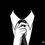# Exponential remainders?

can anyone help me in finding the remainder when $3^{942}$ is divided by 2014?? I dont need answer just help!!!!! please.Note by Gautam Sharma
6 years, 9 months ago

This discussion board is a place to discuss our Daily Challenges and the math and science related to those challenges. Explanations are more than just a solution — they should explain the steps and thinking strategies that you used to obtain the solution. Comments should further the discussion of math and science.

When posting on Brilliant:

• Use the emojis to react to an explanation, whether you're congratulating a job well done , or just really confused .
• Ask specific questions about the challenge or the steps in somebody's explanation. Well-posed questions can add a lot to the discussion, but posting "I don't understand!" doesn't help anyone.
• Try to contribute something new to the discussion, whether it is an extension, generalization or other idea related to the challenge.

MarkdownAppears as
*italics* or _italics_ italics
**bold** or __bold__ bold
- bulleted- list
• bulleted
• list
1. numbered2. list
1. numbered
2. list
Note: you must add a full line of space before and after lists for them to show up correctly
paragraph 1paragraph 2

paragraph 1

paragraph 2

[example link](https://brilliant.org)example link
> This is a quote
This is a quote
    # I indented these lines
# 4 spaces, and now they show
# up as a code block.

print "hello world"
# I indented these lines
# 4 spaces, and now they show
# up as a code block.

print "hello world"
MathAppears as
Remember to wrap math in $$ ... $$ or $ ... $ to ensure proper formatting.
2 \times 3 $2 \times 3$
2^{34} $2^{34}$
a_{i-1} $a_{i-1}$
\frac{2}{3} $\frac{2}{3}$
\sqrt{2} $\sqrt{2}$
\sum_{i=1}^3 $\sum_{i=1}^3$
\sin \theta $\sin \theta$
\boxed{123} $\boxed{123}$

Sort by:

$\phi(2014)=936$

And $\gcd(3,2014)=1$

By Euler-Fermat theorem we have $3^{\phi(2014)}\equiv 3^{936}\equiv 1\mod 2014$

$\Rightarrow 3^{936}\times 3^6\equiv 3^{942}\equiv 729 \mod 2014$

- 6 years, 8 months ago

- 6 years, 8 months ago

Sorry for my mistake I've now edited it. There's no other method to my knowledge. U can look up this theorem in the brilliant wiki.

- 6 years, 8 months ago

@GAUTAM SHARMA Check out Euler's Theorem in the Modular Arithmetic Wiki. That should provide you with explanations about how to approach problems like this.

Staff - 6 years, 8 months ago

Thanks D);.

- 6 years, 8 months ago

NO Problem. BTW IT WAS YOUR QUESTION. I got k=3 but was unable to get remainder.

- 6 years, 8 months ago

I always use Euler's totient function since it reduces exponents into a fathomable number. (Though I still do not know the proof of the theorem, would be great if someone presents one)

- 6 years, 8 months ago

U may find it here

- 6 years, 8 months ago

I want to ask How to read it or interpret this like when we do 12/3=4 , we say when 12 is divided by 3 we get 4 as a quotient.How to read it?

- 6 years, 8 months ago

$12\equiv 1 \mod 11$

is read as "12 congruent to 1 mod 11" or "12 equivalent to 1 mod 11"

- 6 years, 8 months ago